## Introduction

Squeezed spin states1,2,3,4 are such entangled states of collective spins in which the uncertainty of one spin component perpendicular to the mean spin direction is reduced below the standard quantum limit (SQL). They have attracted broad attention due to their applications in high-precision measurements5,6,7,8,9,10 and in the study of many-body entanglement11,12,13,14,15,16,17. Over the past few decades, many studies have been proposed to realize spin squeezing4, mainly involved in Bose-Einstein condensates (BECs) with atom-atom collisions8,9,18,19,20, atom-light interaction systems21,22,23,24,25.

To generate spin squeezing, one direct method is to transfer the squeezing from squeezed light to atomic ensembles, but the transfer efficiency is limited and the degree of spin squeezing depends on the performance of light26,27,28,29. Quantum nondemolition (QND) measurement is also a feasible method to realize spin squeezing, but the acquired squeezing is not deterministic and is degraded by the performance of the detector30,31,32,33,34,35,36,37,38,39,40. Another major type of method to deterministically generate spin squeezing is utilizing squeezing interactions, which typically include the one-axis twisting (OAT) and two-axis twisting (TAT) interactions1,4,41,42,43,44,45,46,47. The OAT interaction, which can be experimentally implemented in some systems including BECs8,9,48, trapped ions49,50,51 and superconducting qubits52,53,54, reaches a squeezing degree  1/N2/3 for N particles. As for the TAT interaction, which is mainly reported in theoretical scheme46,55,56, the squeezing degree is  1/N, approaching to the Heisenberg limit (HL), but implementation of TAT squeezing still remains a challenge. In the atom-light interaction systems, the double-pass squeezing approach based on the Faraday magneto-optical rotation is proposed57, but the squeezing performance  1/N2/5 is far from being satisfactory, which is due to the unwanted residual atom-light entanglement58,59.

Here, we propose a dynamic synthesis scheme to deterministically generate high-degree spin squeezing, without requiring original squeezing interactions. Based on the common non-squeezing interactions, denoted by equation (1), and a periodic rotation pulse sequence, the squeezing interactions can be dynamically synthesized, facilitating a squeezed spin state from an initially uncorrelated state. Both OAT and TAT interactions can be effectively synthesized, which hence leads to a squeezing performance approaching to the HL. Our scheme hence largely enriches the variety of systems that can realize spin squeezing. Furthermore, in our scheme, the strength of the generated interaction is enhanced (faster dynamics or shorter evolution) and the sign of the interaction coefficient is flippable, presenting its capability of realizing the time-reversal dynamics. It hence gives rise to a potential platform to realize technical-noise-immune nonlinear interferometer and study the quantum chaos and information scrambling by measuring the out-of-time-order correlator60,61,62,63,64,65.

## Results

### System model

We consider a universal model with the following interaction Hamiltonian

$${H}_{{{{\rm{int}}}}}=g{S}_{z}{J}_{z},$$
(1)

where g denotes the coupling strength between two subsystems described by the collective spins S and J. This Hamiltonian commonly exists in various systems, such as atom-light interaction systems31,37 and dipole–dipole interaction systems66, as illustrated in Fig. 1(a), (b). Note that in the split BEC system66, there exist intra-species interactions $${S}_{z}^{2}$$ and $${J}_{z}^{2}$$ apart from inter-species interaction SzJz, while in the system of cold or thermal atoms, the intra-species interactions vanish and only inter-species interaction between two subsystems is left, which is the form of equation (1). In the context of atomic ensembles, the operators $${S}_{\mu }=\mathop{\sum }\nolimits_{k = 1}^{{N}_{{{{\rm{s}}}}}}{\sigma }_{{{{\rm{S}}}},\mu }^{(k)}/2$$ and $${J}_{\mu }=\mathop{\sum }\nolimits_{k = 1}^{{N}_{{{{\rm{j}}}}}}{\sigma }_{{{{\rm{J,\mu }}}}}^{(k)}/2\,$$ (μ = x, y, z) define the collective spin components for the two subsystems, with $${\sigma }_{{{{\rm{S}}}},\mu }^{(k)}$$ and $${\sigma }_{{{{\rm{J,\mu }}}}}^{(k)}$$ being the corresponding Pauli matrices for the k-th spin-1/2 (or pseudospin) particle, respectively. As for atom-light interaction system, the operators Jμ denote the Stokes operators, which are related to the differences between the number operators of the photons polarized in different orthogonal bases (see Supplementary Note A). Both of them satisfy the usual SU(2) angular momentum commutation relations [Si, Sj] = iεijkSk and [Ji, Jj] = iεijkJk, where εijk is the Levi-Civita symbol.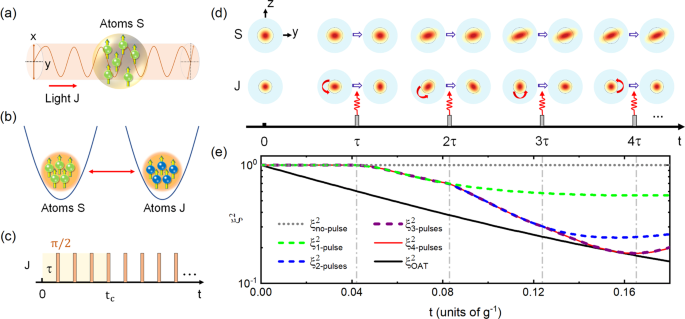Fig. 1: Spin squeezing via dynamic synthesis.

To be clear, we clarify that the studied spin squeezing is focused on one subsystem (e.g., S), which contains the contribution of the other subsystem J through their interaction. This is mainly based on the fact that the squeezing of one subsystem is usually easier to be implemented than that of the united system (S + J) in practical application, especially for the case with quite different subsystems. For example, for atom-light interaction systems, the polarization of photons and collective atomic spin can not be naturally combined to serve as the whole resources of squeezing (see Supplementary Note H). More specifically, in the model Hamiltonian (1), intra-species interaction ($${S}_{z}^{2}\,{{{\rm{or}}}}\,{J}_{z}^{2}$$) in both subsystems is absent and the atoms hence maintain approximately uncorrelated following the evolution based on the interaction Hamiltonian (1), if they are initially uncorrelated. An effective intra-species interaction always occurs as a high-order effect from the trivial inter-species interaction (SzJz), i.e., subsystem J carries the interaction among atoms in subsystem S, as an auxiliary force carrier.

To amplify such a high-order effect and eliminate the influence of low-order interaction, we put forward a controlled pulse scheme, which contains several rotation pulses described by the following Hamiltonian

$${H}_{{{{\rm{rot}}}}}={{{\Omega }}}_{1}(t){J}_{x}+{{{\Omega }}}_{2}(t){S}_{x}.$$
(2)

It leads to a spin rotation of angle $${\theta }_{1,2}=\int\nolimits_{{t}_{0}}^{{t}_{0}+{{\Delta }}t}{{{\Omega }}}_{1,2}(t)dt$$ around x axis over a short pulse duration Δt starting from t0 for subsystem J and S, respectively. Their corresponding rotation operators are defined as $${R}_{{{{\rm{J}}}}}(\theta )={{{{\rm{e}}}}}^{-{{{\rm{i}}}}\theta {J}_{x}}$$ and $${R}_{{{{\rm{S}}}}}(\theta )={{{{\rm{e}}}}}^{-{{{\rm{i}}}}\theta {S}_{x}}$$.

### Generation of effective OAT squeezing

First, we propose a pulse scheme to synthesize the effective OAT squeezing. In this case, only periodic π/2 rotation pulses are required to implement on the subsystem J with equal pulse separation τ. Each period contains four segments of free evolution and four π/2 rotation pulses, as sketched in Fig. 1(c). Neglecting the pulse duration Δt, the evolution operator for a single period tc 4τ is given by

$${U}_{1}(4\tau )={\left[{R}_{{{{\rm{J}}}}}\left(\frac{\pi }{2}\right)U(\tau )\right]}^{4},$$
(3)

where U(τ) = $${{{{\rm{e}}}}}^{-{{{\rm{i}}}}g{S}_{z}{J}_{z}\tau }$$ is the free evolution operator under the interaction Hamiltonian (1). The evolution operator can be rewritten as

$$\begin{array}{ll}{U}_{1}(4\tau )={{{{\rm{e}}}}}^{{{{\rm{i}}}}g{S}_{z}{J}_{y}\tau }{{{{\rm{e}}}}}^{{{{\rm{i}}}}g{S}_{z}{J}_{z}\tau }{{{{\rm{e}}}}}^{-{{{\rm{i}}}}g{S}_{z}{J}_{y}\tau }{{{{\rm{e}}}}}^{-{{{\rm{i}}}}g{S}_{z}{J}_{z}\tau }\equiv \mathop{\prod }\limits_{k=1}^{4}{{{{\mathcal{U}}}}}_{k},\end{array}$$
(4)

as a product of four unitary operators. According to the Baker-Campbell-Hausdorff formula, omitting the higher-order terms $${{{\mathcal{O}}}}({(g\tau )}^{2})$$, the evolution operator is approximately derived as $${U}_{{{{\rm{1}}}}}(4\tau )\simeq \exp (-{{{\rm{i}}}}{g}^{2}{S}_{z}^{2}{J}_{x}{\tau }^{2})$$. When the subsystem J initially stays in a coherent state (Nj uncorrelated particles) polarized along the x axis, i.e., the eigenstate of Jx with the eigenvalue Nj/2, the effective Hamiltonian can be obtained as (see Supplementary Note B),

$${H}_{{{{\rm{eff}}}}}^{{{{\rm{OAT}}}}}={\chi }_{{{{\rm{eff}}}}}{S}_{z}^{2},$$
(5)
$${\chi }_{{{{\rm{eff}}}}}=\frac{1}{8}{N}_{{{{\rm{j}}}}}{g}^{2}\tau .$$
(6)

We hence obtain an effective OAT Hamiltonian, giving rise to squeezing dynamics in subsystem S.

The understanding of the pulse scheme is as follows. Subsystem J plays the role of an intermediary to induce the intra-species interaction in subsystem S. This process is analogous to the effective electron-electron interaction mediated by the phonons (lattice vibrations) in condensed matter physics, such as the superconducting Bardeen-Cooper-Schrieffer theory, which can be treated by the Frohlich-Nakajima transformation67,68. The product of the first three evolution operators, $${{{{\mathcal{U}}}}}_{3}{{{{\mathcal{U}}}}}_{2}{{{{\mathcal{U}}}}}_{1}$$, in each period constitutes an equivalent Frohlich-Nakajima transformation (FNT)67,68, representing that we apply unitary transformation on the evolution operator. According to the operator expansion formula

$${{{{\rm{e}}}}}^{S}H{{{{\rm{e}}}}}^{-S}=H{{{\rm{+}}}}[S,H]+\frac{1}{2!}[S,[S,H]]+...,$$
(7)

the product $${{{{\mathcal{U}}}}}_{3}{{{{\mathcal{U}}}}}_{2}{{{{\mathcal{U}}}}}_{1}$$ can be expanded as

$${{{{\rm{e}}}}}^{S}{{{{\rm{e}}}}}^{-{{{\rm{i}}}}H\tau }{{{{\rm{e}}}}}^{-S}={{{{\rm{e}}}}}^{-{{{\rm{i}}}}\left\{H{{{\rm{+}}}}[S,H]+\frac{1}{2!}[S,[S,H]]+...\right\}\tau },$$
(8)

where H = gSzJy denotes the Hamiltonian in the second segment in each period and S = igSzJzτ denotes the unitary transformation, arising from the real unitary evolution. The proper form of S can make the commutator [S, H] contains squeezing term $${g}^{2}\tau {J}_{x}{S}_{z}^{2}$$, leading to the squeezing of subsystem S. In order to eliminate the first-order term, i.e., the residual linear interacting term in equation (8), we need to add another segment of real unitary evolution eiHτ, i.e., $${{{{\mathcal{U}}}}}_{4}$$, such that

$$\begin{array}{ll}{{{{\rm{e}}}}}^{{{{\rm{i}}}}H\tau }{{{{\rm{e}}}}}^{S}{{{{\rm{e}}}}}^{-{{{\rm{i}}}}H\tau }{{{{\rm{e}}}}}^{-S}={{{{\rm{e}}}}}^{-{{{\rm{i}}}}\left\{[S,H]+\frac{1}{2!}[S,[S,H]]+...\right\}\tau } \approx {{{{\rm{e}}}}}^{-{{{\rm{i}}}}{g}^{2}{\tau }^{2}{J}_{x}{S}_{z}^{2}},\end{array}$$
(9)

for which the higher-order terms are ignored. This corresponds to the evolution operator of squeezing interaction, indicating that the second-order perturbation leads to the OAT squeezing interaction $${S}_{z}^{2}$$. In each period, subsystem J hence serves as an auxiliary system for “information storage, processing and feedback”, which stores the quantum state of subsystem S, and transfers the information back to subsystem S. (see Supplementary Note D for details). It is noticed that no additional information about subsystem S is carried by subsystem J after each complete period, since the output state of J in each period remains an uncorrelated coherent spin state the same as its input, as shown in Fig. 1(d). Note that in the reported cavity feedback mechanism7,69, the cavity field plays the similar role of subsystem J in our scheme. However, we should point out that our scheme is general to extensive systems as long as the interaction has the form of coupling two collective (pseudo-)spins, rather than being limited in the specific atom-light interaction systems.

To verify the validity of the effective OAT Hamiltonian (5), we numerically investigate the evolutions of the quantum state in the full Hilbert space within the first period of our scheme. It is demonstrated by their representations (Husimi Q function after partially tracing out one of the subsystems) on the generalized Bloch spheres in Fig. 1(d). The overall quasiprobability distribution of subsystem J rotates 90 degrees after each π/2 pulse. While as for subsystem S, it is continuously squeezed, representing the OAT dynamics. The degree of spin squeezing is usually quantified by the squeezing parameter $${\xi }^{2}=4{({{\Delta }}{S}_{\perp })}_{\min }^{2}/{N}_{{{{\rm{s}}}}}$$ for Kitagawa and Ueda criterion1, or $${\xi }_{{{{\rm{R}}}}}^{2}={N}_{{{{\rm{s}}}}}{({{\Delta }}{S}_{\perp })}_{\min }^{2}/| \langle {{{\bf{S}}}}\rangle {| }^{2}$$ for Wineland criterion2,3, where $${({{\Delta }}{S}_{\perp })}_{\min }^{2}$$ is the minimum of the fluctuation $${({{\Delta }}{S}_{\perp })}^{2}=\langle {S}_{\perp }^{2}\rangle -{\langle {S}_{\perp }\rangle }^{2}$$ for the spin component perpendicular to the mean spin direction and S is the mean spin length. In Fig. 1(e) the evolution of the squeezing parameters for different cases are exhibited. Without rotation pulses, the squeezing parameter keeps ξ2 = 1 (gray dotted curve), denoting that the SzJz type interaction alone cannot dynamically introduce spin squeezing starting from initially uncorrelated states as we previously discussed. When rotation pulses are applied, the squeezing parameter decreases, indicating spin squeezing. At the end of the first period with four π/2 rotation pulses, the squeezing parameter (red solid curve) touches the curve for the OAT squeezing (black solid curve) governed by the Hamiltonian (5). It is noted that only one or two rotation pulses can also lead to squeezing (dashed curves), while the squeezing degree cannot reach as high as the OAT results.

The optimal squeezing parameter (for Kitagawa and Ueda criterion) and the corresponding squeezing time for the synthesized effective OAT interaction are derived as

$${\xi }_{\min }^{2}\simeq \frac{1}{2}{\left(\frac{{N}_{{{{\rm{s}}}}}}{3}\right)}^{-\frac{2}{3}},{{\mbox{}}}{t}_{\min }\simeq \frac{8\times {3}^{1/6}}{{g}^{2}\tau {N}_{{{{\rm{j}}}}}{N}_{{{{\rm{s}}}}}^{2/3}}.$$
(10)

Their validity for both Kitagawa parameter ξ2 and Wineland parameter $${\xi }_{{{{\rm{R}}}}}^{2}$$ is demonstrated by the exact numerical simulation as compared in Fig. 2, including the evolution of squeezing parameter and the N scaling about the optimal squeezing parameter and squeezing time. The power-law scalings of both $${\xi }_{\min }^{2}$$ and $${t}_{\min }$$ with respect to subsystem S are the same as the OAT case, i.e., $${\xi }_{\min }^{2}\propto 1/{N}_{{{{\rm{s}}}}}^{2/3}$$ and $${t}_{\min }\propto 1/{N}_{{{{\rm{s}}}}}^{2/3}$$. Here we consider a fixed τ, since it is an artificially controlled parameter, while the restricted condition for τ, i.e., Eq. (32) in Supplementary Note C, is just a sufficient condition. It notes that the optimal squeezing parameter for subsystem S is independent of subsystem J, since they become uncorrelated after each pulse sequence period. As for the optimal squeezing time, there is an additional power-law scaling with respect to Nj, i.e., $${t}_{\min }\propto 1/{N}_{{{{\rm{j}}}}}$$, as shown in Fig. 2(c), due to the dependence of Nj on the effective coupling strength. It suggests that an additional degree of freedom to accelerate the arrival of optimal spin squeezing is available, resulting in preceding the conventional OAT squeezing that is usually limited in a single atomic ensemble. In addition, the squeezing of one subsystem for our scheme performs better than that of the united system under free evolution of the Hamiltonian (1), when the particle numbers of two subsystems are quite different (see Supplementary Note H).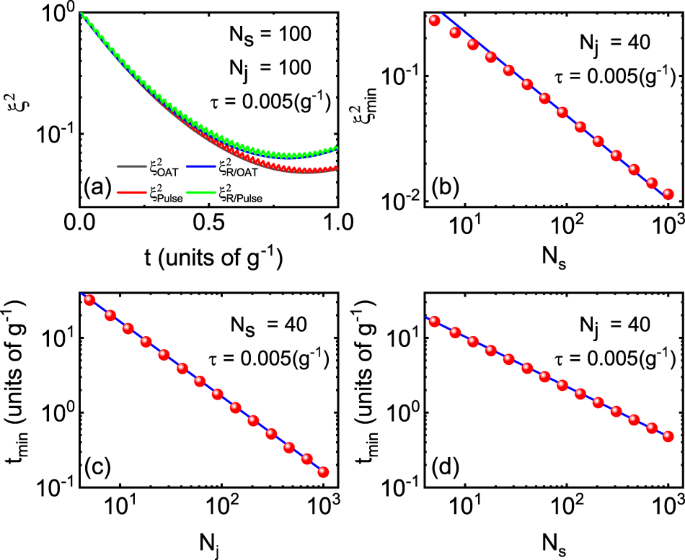Fig. 2: Dynamic synthesis of OAT squeezing.

Furthermore, our scheme is also utilizable for a fairly small number of pulses. A large number of pulses, corresponding to sufficiently small pulse separation τ, can guarantee the validity of our pulse scheme almost all the time, as shown in Fig. 3(a), which takes the effective OAT scheme with 200 pulses as an example. However, if we only focus on achieving the optimal squeezing, the pulse separation is not necessary to be tiny, and thus the pulse number can be reduced. As plotted in Fig. 3(b)–(d), if we reduce the pulse number, the oscillation amplitude of the squeezing parameter increases, but the optimal squeezing is still achievable. This is because only the time instants at the end of each period are important. From Fig. 3(d) we can find that even for as few as 4 pulses, it is sufficient to reach the optimal squeezing. Moreover, fewer pulse number (larger pulse separation τ) corresponds to stronger interaction strength, and thereby shorter squeezing time, as indicated from equations (10) and (13), which is also verified by comparing the optimal squeezing time in Fig. 3(a)–(d). Systematically, Fig. 3(e), (f) further confirm the properties of our scheme.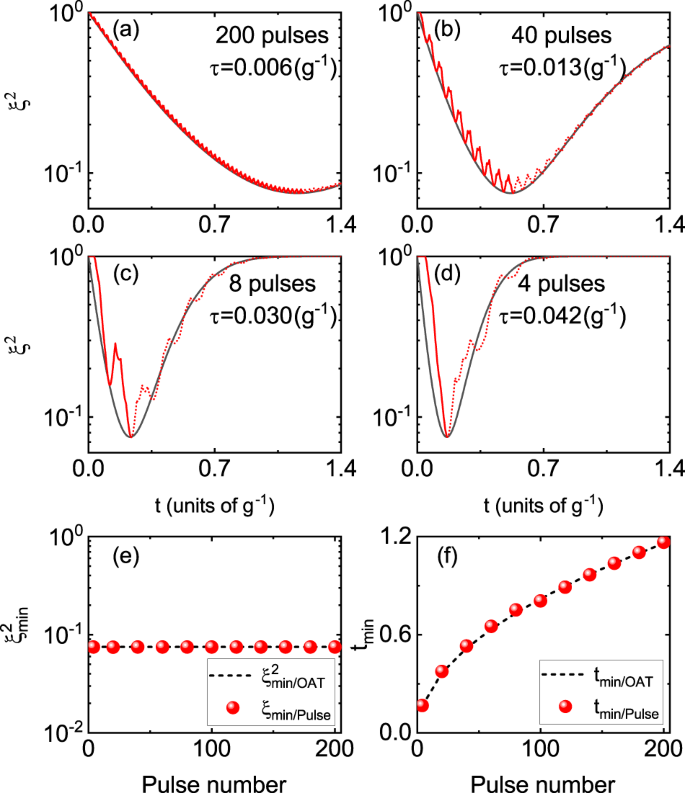Fig. 3: Influence of pulse numbers on the squeezing parameter ξ2.

It is also noticed that both the interaction strength and its sign of such an effective OAT Hamiltonian (5) are tunable. On one hand, the strength increases as the subsystem J enlarges, due to χeffNj. On the other hand, the sign of the interaction coefficient can be flipped by simply alternating all the π/2 rotation pulses to −π/2 (or 3π/2) rotation pulses, yielding χeff = −Njg2τ/8 and time-reversal dynamics. The time-reversal untwisting dynamics is of importance to construct an interaction-based readout echo60,61, which is often applied to uncover the metrological usefulness of entangled non-Gaussian state and overcome the problem of limited atomic number resolution70,71.

### Generation of effective TAT squeezing

The squeezing performance can be further enhanced to the HL, since a more complicated pulse sequence is capable of synthesizing the TAT interaction. On this purpose, inspired by the idea in ref. 42 for which the squeezing axes are alternatively changed during evolution, additional control pulses on S are required since TAT interaction demands two squeezing axes, e.g., z-axis and y-axis for $${S}_{z}^{2}-{S}_{y}^{2}$$. This improved scheme is illustrated in Fig. 4(a). We apply two series of rotation pulses on both subsystems S and J: each period contains four π/2 rotation pulses and four − π/2 rotation pulses acting on subsystem J with equal pulse separation τ, added with a pair of π/2 rotation pulses acting on subsystem S with pulse separation 4τ. The evolution operator for a single period Tc 8τ is given by

$${U}_{{{{\rm{2}}}}}(8\tau )={R}_{{{{\rm{S}}}}}\left(\frac{\pi }{2}\right){\left[{R}_{{{{\rm{J}}}}}\left(-\frac{\pi }{2}\right)U(\tau )\right]}^{4}{R}_{{{{\rm{S}}}}}\left(-\frac{\pi }{2}\right){\left[{R}_{{{{\rm{J}}}}}\left(\frac{\pi }{2}\right)U(\tau )\right]}^{4}.$$
(11)

The resulted effective TAT Hamiltonian is straightforward as following the derivations of the effective OAT Hamiltonian (see Supplementary Note I for details), obtained as

$${H}_{{{{\rm{eff}}}}}^{{{{\rm{TAT}}}}}=\frac{1}{2}{\chi }_{{{{\rm{eff}}}}}({S}_{z}^{2}-{S}_{y}^{2}).$$
(12)

Here, the effective interaction coefficient of TAT squeezing is half the case of effective OAT squeezing, i.e., χeff/2, while the sign of this coefficient is also flippable by merely changing the phase or areas of the rotation pulses on subsystem J. Since the sign of the obtained effective interaction coefficient χeff for OAT can be changed, the pulse sequence on subsystem S of realizing the effective TAT squeezing is simply equally spaced pulses, much easier to realize compared with that in ref. 42, as shown in Fig. 4(a). Furthermore, the effective interaction coefficient of TAT squeezing in our scheme (χeff/2) is larger than that in ref. 42 (χeff/3), which is more favorable for achieving the optimal spin squeezing within shorter time.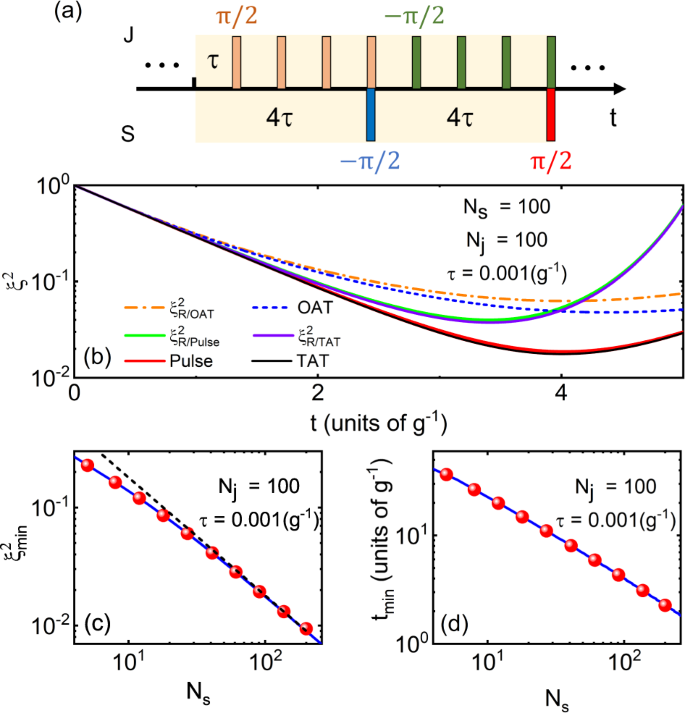Fig. 4: Dynamic synthesis of TAT squeezing.

The optimal squeezing parameter and the corresponding squeezing time for the synthesized TAT interaction is approximate to

$${\xi }_{\min }^{2}\simeq \frac{1.8}{{N}_{{{{\rm{s}}}}}},{{\mbox{}}}{t}_{\min }\simeq \frac{8{{\mathrm{ln}}}\,(4{N}_{{{{\rm{s}}}}})}{{g}^{2}\tau {N}_{{{{\rm{j}}}}}{N}_{{{{\rm{s}}}}}}.$$
(13)

The validity of the effective TAT Hamiltonian (12) is verified by the exact numerical simulation in Fig. 4(b)–(d). The numerical results of the pulse scheme agrees with the effective TAT squeezing, dramatically outperforming the OAT scheme. The power-law scalings of both $${\xi }_{\min }^{2}$$ and $${t}_{\min }$$ with respect to Ns are also the same as the TAT case, as shown in Fig. 4(c), (d). It reveals that the spin squeezing with measurement sensitivity approaches to the HL and its corresponding required time is much less than that of the optimal OAT squeezing. In addition, analogous to the OAT squeezing discussed above, a relatively small number of pulses can also achieve the best attainable squeezing that approaches to the optimal TAT squeezing (see Supplementary Note K for details).

## Discussion

Our scheme is robust to various noises including fluctuations of pulse areas and pulse separations. On one hand, we carry out the numerical simulation by adding Gaussian stochastic noises, i.e., assuming the fluctuating pulse areas or pulse separations are subject to Gaussian distribution with the standard deviation about five percent of the average value. The squeezing parameters of 500 independent simulations under the two types of noises are respectively shown in Fig. 5(a), (b). On the other hand, we analyze the influence of error size on the best attainable squeezing, as shown in Fig. 5(c), (d). For different pulse area or pulse separation error size, ranging from –5% to 5%, the best attainable squeezing can almost achieve the optimal squeezing of the effective OAT dynamics. Thus, it reveals that the squeezing still remains under the influence of noises, indicating the robustness of the pulse scheme. In addition, we investigate the dependence of robustness to imperfect rotations on system size. For larger particle numbers, the robustness still remains and results show that it is essentially size-independent with respect to the system (see Supplementary Note L for details).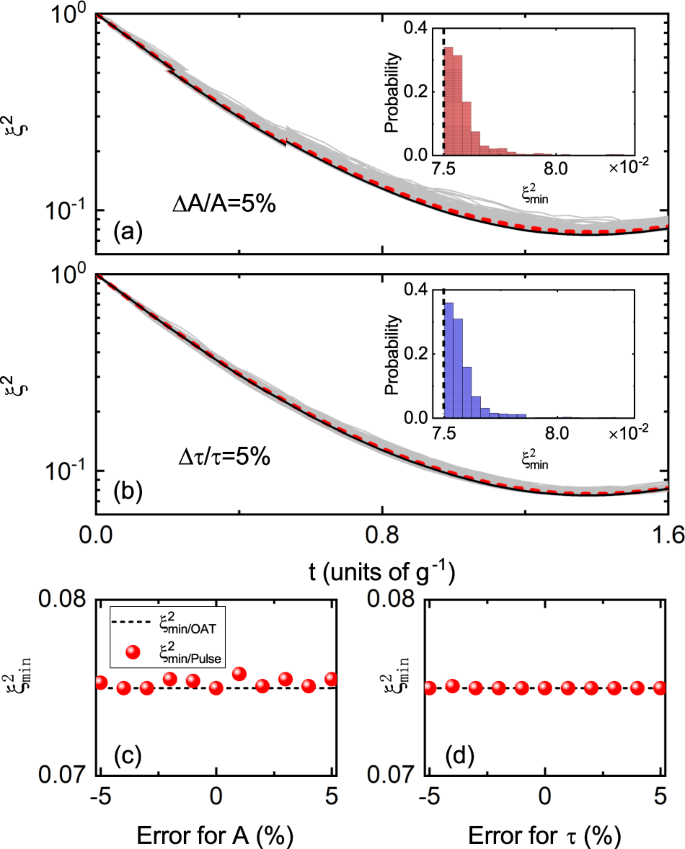Fig. 5: Noise analysis for our scheme.

Many efforts have been made to study the decoherence of spin squeezing72,73. Particularly, an open-source library in PYTHON, the Permutational-Invariant Quantum Solver, has been developed to study different types of decoherence in open quantum system and address the robustness of various collective phenomena, including spin squeezing74. For example, in BECs, particle losses (or atomic decay) may be the major source of decoherence75,76. However, since the particle losses are the intrinsic attributes of the systems themselves instead of being caused by our pulse scheme, the particle losses have the similar influence for our scheme compared with the situation without pulses.

We note that the effective OAT or TAT squeezing can also be realized in other systems, such as nitrogen vacancy (NV) centers coupled to mechanical modes77,78,79. In NV centers, spin dephasing degrades the squeezing77, while it can be solved by dynamical decoupling80. Our scheme is applicable in NV centers and is compatible with dynamical decoupling, since the essence of dynamical decoupling is also applying a sequence of pulses that repetitively flip the state of the system.

In summary, we have presented a universal scheme to dynamically synthesize spin squeezing based on non-squeezing interactions and a series of rotation pulses. The non-squeezing interaction can be effectively transformed into squeezing interactions, either OAT interaction or TAT interaction. The effective interaction strengths are strongly enhanced due to the collective effect and their signs are also flippable, being either positive or negative, which depend on the controlled phase or area of the rotation pulses. This feature largely extends the parameter space for experiments, and enables the construction of time-reversal nonlinear interferometer with high measurement precision. The generated spin squeezing can ultimately reach the HL with measurement precision  1/Ns. Meanwhile, our scheme is demonstrated to be robust to fluctuating pulse areas and pulse separations. Moreover, a small number of pulses remains valid for achieving the optimal squeezing. This study provides the opportunity for realizing Heisenberg-limited spin squeezing in a large number of non-squeezing systems, which is significant for quantum metrology and quantum information science.

## Methods

### Calculation of the quasiprobability distribution

First, we introduce the calculation of the quasiprobability distribution, the Husimi Q function, for each subsystem. For the sake of description, we denote the quasiprobability distribution as Q(θs, ϕs) for subsystem S and Q(θj, ϕj) for J. The time-dependent quantum state of the whole system is written as $$\left|\psi (t)\right\rangle$$ at t. Since the dimension of Hilbert space is (Ns + 1)(Nj + 1), we project the quantum state into the product state of two arbitrary coherent spin states, i.e., $$\left|{\theta }_{{{{\rm{s}}}}},{\phi }_{{{{\rm{s}}}}}\right\rangle \bigotimes \left|{\theta }_{{{{\rm{j}}}}},{\phi }_{{{{\rm{j}}}}}\right\rangle$$, whose Hilbert space dimensions are Ns + 1 and Nj + 1, respectively. And the quasiprobability is obtained as $$P=| \left\langle {\theta }_{{{{\rm{s}}}}},{\phi }_{{{{\rm{s}}}}}\right|\left\langle {\theta }_{{{{\rm{j}}}}},{\phi }_{{{{\rm{j}}}}}| \psi (t)\right\rangle {| }^{2}$$. Then we integrate the value of P with respect to one pair of (θ, ϕ), which is equivalent to the partial trace of the pure quantum state $$\left|\psi (t)\right\rangle$$, obtaining the quasiprobability distribution for one subsystem, e.g., S

$$Q({\theta }_{{{{\rm{s}}}}},{\phi }_{{{{\rm{s}}}}})=\int\nolimits_{0}^{\pi }\int\nolimits_{0}^{2\pi }Pd{\theta }_{{{{\rm{j}}}}}d{\phi }_{{{{\rm{j}}}}}.$$
(14)

It gives a representation of each subsystem on a generalized Bloch sphere with a polar angle θ and an azimuthal angle ϕ. In the numerical calculation, for convenience, we replace the integral with a summation about these two angles in a discrete space.

### Calculation of the squeezing parameter

Second, we give the brief process of calculation of the squeezing parameter $${\xi }^{2}=4{({{\Delta }}{S}_{\perp })}_{\min }^{2}/{N}_{{{{\rm{s}}}}}$$1, as mentioned previously. To calculate $${({{\Delta }}{S}_{\perp })}_{\min }^{2}$$, we fix a direction in the perpendicular plane of the mean spin direction, might as well being denoted as n. Therefore, in the perpendicular plane, $${({{\Delta }}{S}_{\perp })}^{2}$$ is a function of the angle α, which is defined as the included angle between an arbitrary direction and the direction of n. By making the derivative of $${({{\Delta }}{S}_{\perp })}^{2}$$ with respect to α equal to zero, i.e., $$d{({{\Delta }}{S}_{\perp })}^{2}/d\alpha =0$$, we can obtain the minimum value of the fluctuation $${({{\Delta }}{S}_{\perp })}_{\min }^{2}$$ with the optimal squeezing angle αopt. More detailed calculation is also given in ref. 81.

### Evolution of the squeezed spin states

Besides, since our calculation is based on the quantum state $$\left|\psi (t)\right\rangle$$ at t, we give the description of numerical procedure of calculating $$\left|\psi (t)\right\rangle$$. We divide the whole evolution operator U(t) into many small equal parts U(δt), denoting a segment of free evolution under the Hamiltonian (1) for a very short time δt (δtτ). Given the initial coherent spin state $$\left|\psi \right\rangle$$, we apply the individual evolution operator U(δt), together with the rotation operators RS( ± π/2) or RJ( ± π/2) at appropriate time instants, repeatedly on the evolved state and can approximately obtain the time-dependent state. As a result, the evolution of quantum state and squeezing parameter are obtained.